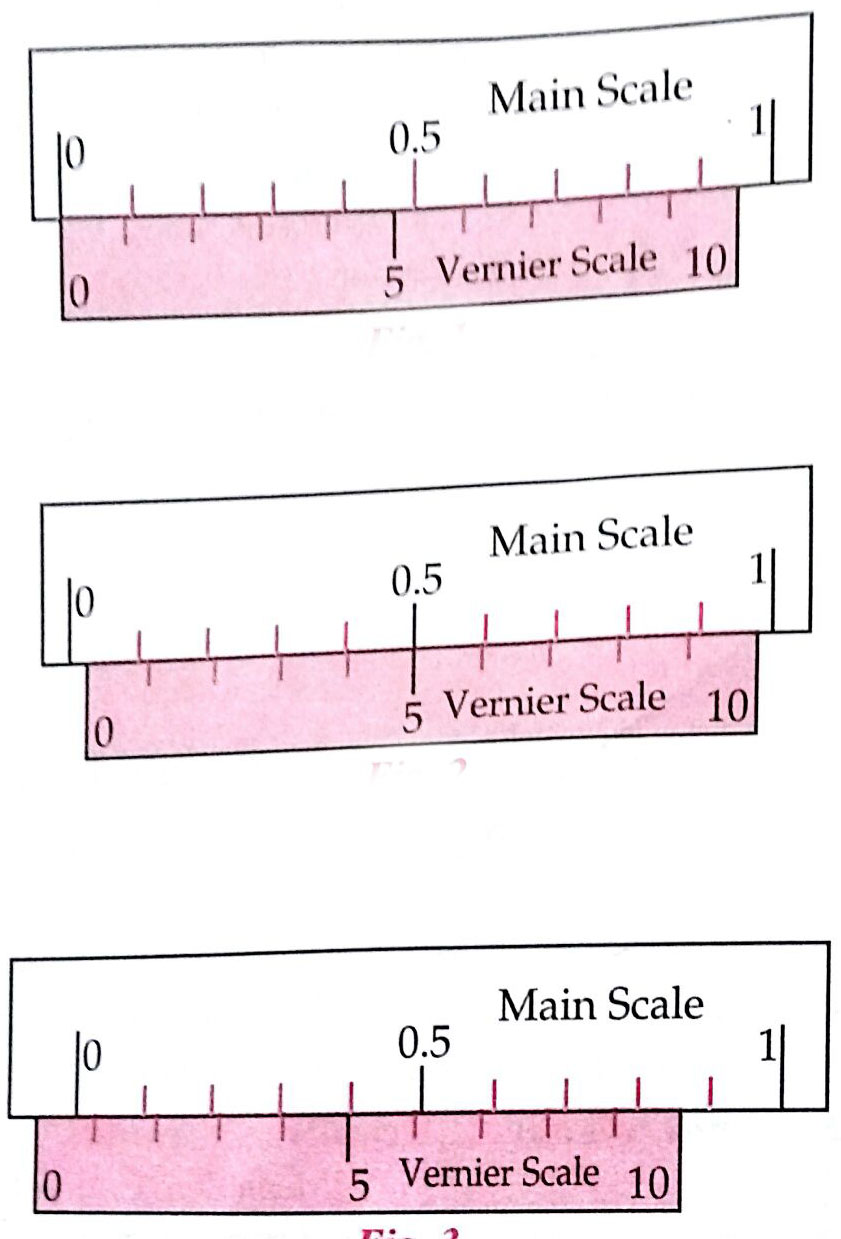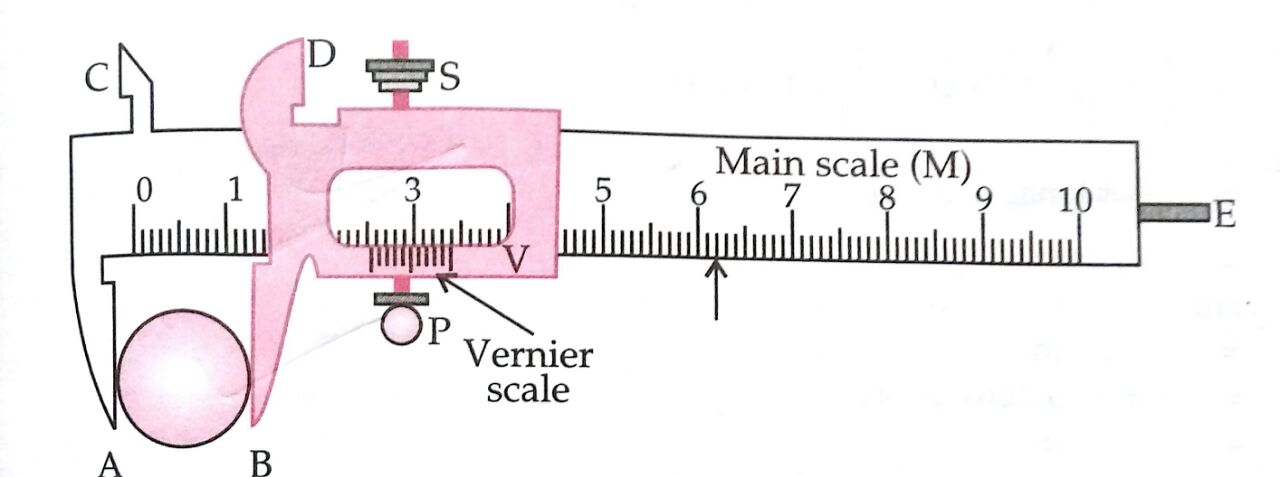# Two full turns of the circular scale of a screw gauge cover a distance of 1 mm on its main scale. The total number of divisions on the circular scale is 50. Further, it is found that the screw gauge has a zero error of – 0.03 mm. While measuring the diameter of a thin wire, a student notes the main scale reading of 3 mm and the number of circular scale divisions in line with the main scale as 35. The diameter of the wire is Option 1) 3.38 mm Option 2) 3.32 mm Option 3) 3.73 mm Option 4) 3.67 mm.

P Prateek Shrivastava

As we learnt in

To measure the diameter of small spherical cylindrical body using Vernier Callipers -Vernier Constant

= 1 Main scale division - 1 V.S. Division

V.C= 1 M.S.D - 1 V.S.D

- wherein

N= Nth division

Observations:

1.    Vernier constant (least count) of the Vernier Callipers:

1 M.S.D. = 1 mm

10 vernier scale divisions = 9 main scale divisions

i.e.     10 V.S.D. = 9 M.S.D.

1 V.S.D.   M.S.D.

Vernier Constant (L.C.) = 1 M.S.D. - 1 V.S.D. = 1 M.S.D.  M.S.D.

2.    Zero error: (i) ........ cm (ii) ............cm (iii) ..........cm

Mean Zero Error (e) = ............ cm

Mean Zero Correction (c) = - (Mean Zero Error)

= .......... cm

Diameter = M.S.R + C.S.R x LC + Z.E

Option 1)

3.38 mm

This option is correct

Option 2)

3.32 mm

This option is incorrect

Option 3)

3.73 mm

This option is incorrect

Option 4)

3.67 mm.

This option is incorrect

Exams
Articles
Questions